### Home > INT2 > Chapter 12 > Lesson 12.2.4 > Problem12-145

12-145.
1. A map of an island is shown at right. Each unit of length on the grid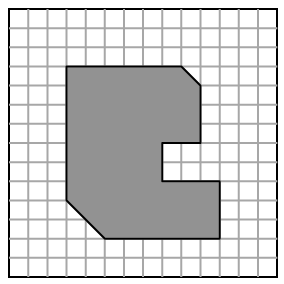represents 32 feet. Homework Help ✎

1. Determine the actual dimensions of the island (the overall width and length).

2. Calculate the area of the shape at right and the actual area of the island.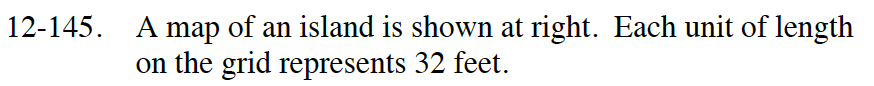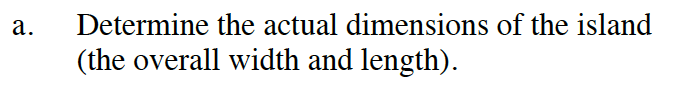What is the overall width and length in square units?

9 square units by 8 square units
Now solve for the actual dimensions of the island.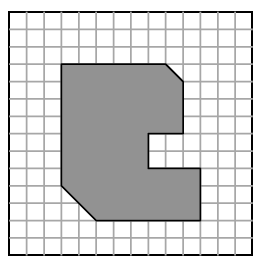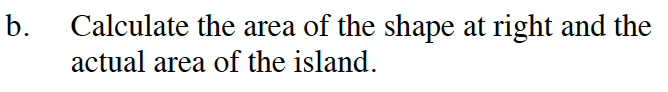Use the answer to hint (a) to solve the first part of the question.

area of island = 60,928 square feet